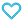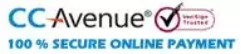# Rank Up Physics JEE Main & Advanced Mechanics Volume-1

425.00

• Paper Book

Discount offer on this book in a bundle, click to view

MTG’s Rank Up Physics for JEE Main & Advanced is a Physics book series comprising 5 books. The series is created by Physics experts to ensure that a JEE (M+A) aspirant can cover the Physics syllabus of JEE exam from all dimensions. The book is an excellent companion for students who wish to learn physics from scratch.
To develop a strong understanding of concepts, the chapters are well explained in a simple language and along with solved intext examples and fundamental questions. The exercises and previous years’ questions in each volume are well equipped with answer keys and explanations so that the student can self-evaluate their understanding of concepts.

149 in stock

Qty:Add to WishlistMTG’s Rank Up Physics is an exhaustive book series for the preparation of the Physics syllabus of JEE Main & Advance. The book series includes Five books namely-
• Mechanics Volume 1 (Covers: Basic Mathematics, Unit, Dimensions, and Errors, Vectors, Motion, Projectile Motion, Laws of Motion, Work, Energy and Power, Circular Motion, and Centre Of Mass and Conservation of Momentum)
• Mechanics Volume 2 (Covers: Rotational Motion, Gravitation, Elasticity, Fluid Mechanics, Simple Harmonics)
• Waves And Thermodynamics (Covers: Wave Motion, Superposition of waves, Sound Waves, Thermal Properties and Kinetic Theory, Thermodynamics and Heat Transfer)
• Electricity And Magnetism (Covers: Electrostatics, Capacitors, Electric Current, Magnetic Force, and Magnetic Field, Electromagnetic Induction, and Alternating Current)
• Optics And Modern physics (Covers: Geometrical Optics, Wave Optics, Atomic Physics, Nuclear Physics)
The uniqueness of this series lies in the following fact:
• Complete coverage of syllabus.
• Simplified presentation of complex Physics concepts.
• Numerous solved examples, questions & numerical for each chapter.
• Variety of questions like – single correct answer type, multiple correct answer type, passage-based, matrix match, integer type, etc. to prepare you for both JEE Main & Advanced.
• Previous 2 years’ (2020 & 2021) questions of JEE Main and JEE Advanced with detailed solutions.

Table of Content:
• Unit 1: Basic Mathematics
• Differentiation
• Rules for Differentiation
• Applications of Derivative
• Integration
• Application of Integration
• Unit 2: Units, Dimensions and Errors
• Introduction
• Units
• Definitions of Fundamental or Base Units (in SI System)
• Supplementary Units
• Derived Units
• Dimensions
• Uses of Dimensions
• Limitations of the Dimensional Method
• Significant Digits
• Rules for Determining the Number of Significant Digits in a Number
• Significant Figures in Algebraic Operations
• Rules for Rounding off Digits
• Errors
• •Propagation of Errors
• Exercise
• Unit 3: Vectors
• Vectors and Scalar
• Representation of a Vector
• Types of Vector
• Angle between Vectors
• Equilibrium of Three Coplanar Vectors
• Multiplication of a Vector by a Scalar
• Subtraction of Vectors
• Resolution of Vector
• Unit vector
• Vector Multiplication
• Exercise
• Unit 4: Motion
• Introduction
• Motion
• Graphs
• Uniformly Accelerated Motion
• Calculus Based Analysis
• Motion Under Gravity
• Relative Motion
• Exercise
• Unit 5: Projectile Motion
• Two-Dimensional Motion
• Equation of Trajectory
• Radius of Curvature (r) of the Trajectory
• Total Displacement and Total Distance
• Projectile Motion
• Projectile Projected from a Moving Frame of Reference
• Projectile Projected from Top of a Tower
• Projectile Motion on Inclined Plane
• Exercise
• Unit 6: Laws of Motion
• Introduction
• Newton’s Laws of Motion
• Weight of a Body
• Free Body Diagram (F.B.D.)
• Normal Force
• Tension in a String
• Equilibrium
• Pulleys
• Monkey Climbing on a String
• Constraint Motion
• Frame of Reference
• Pseudo Force
• Friction
• Two-Blocks System
• Three-Blocks Systems
• Exercise
• Unit 7: Work, Energy and Power
• Work
• Work done by a Constant Force
• Work done by Multiple Forces
• Work done by Variable Force
• Graphical Interpretation of Work Done
• Work Done due to Internal Forces
• Work Depends on the Frame of Reference
• Work-Energy Theorem
• Conservative and Non-conservative Forces
• Energy
• Relation between Potential Energy and Conservative Force
• Translatory Equilibrium
• Ideal Spring
• Cutting of Springs
• Combination of Spring (Massless and Ideal)
• Type of Deformation in Springs
• Power (A Scalar Quantity)
• Exercise
• Unit 8: Circular Motion
• Circular Motion
• Kinematics of Circular Motion
• Tangential and Normal Acceleration
• Dynamics of Circular Motion
• Uniform Circular Motion (UCM)
• Conical Pendulum
• Non-Uniform Circular Motion
• Vertical Circular Motion
• Circular Turnings and Banking of Roads
• Centrifugal Force
• Condition of Toppling of a Vehicle on a Circular Road
• The Spinning Terror
• Motion on the Outer Surface of a Fixed Smooth Sphere
• Exercise
• Unit 9: Centre of Mass and Conservation of Momentum
• Centre of Mass
• Centre of Mass of Two Particles System
• Centre of Mass of Many Particles System
• Centre of Mass and Centre of Gravity
• Change in Position of Centre of Mass
• Centre of Mass of a Continuous Mass Distribution
• Motion of Centre of Mass
• Analysis of Motion of Centre of Mass
• Explosion
• Centre of Mass as a Frame of Reference
• Collision
• Classification of Collisions
• Impulse and Momentum
• Oblique Collision
• Variable Mass
• Exercise